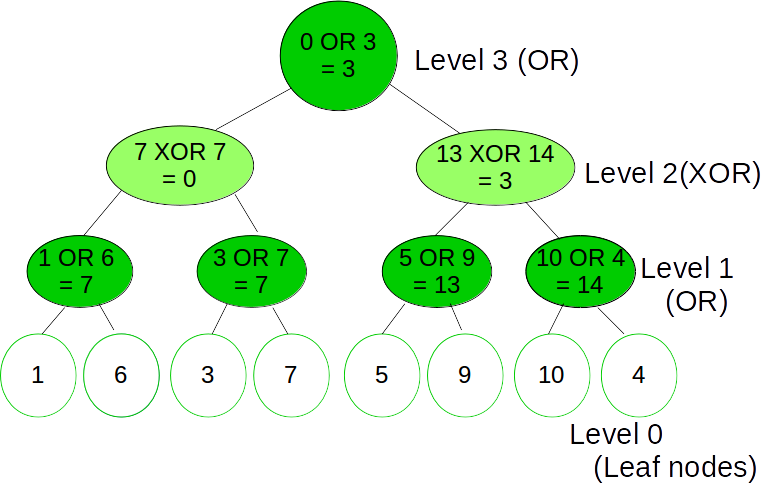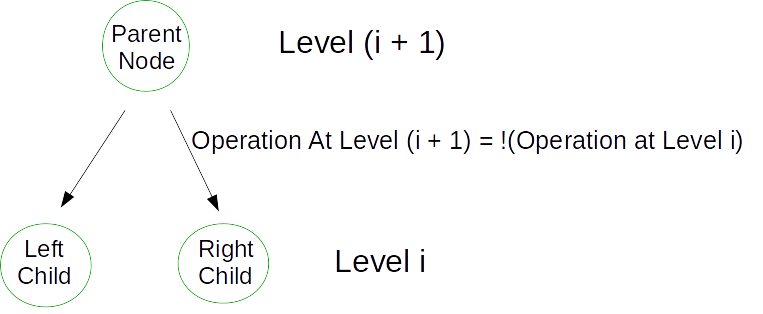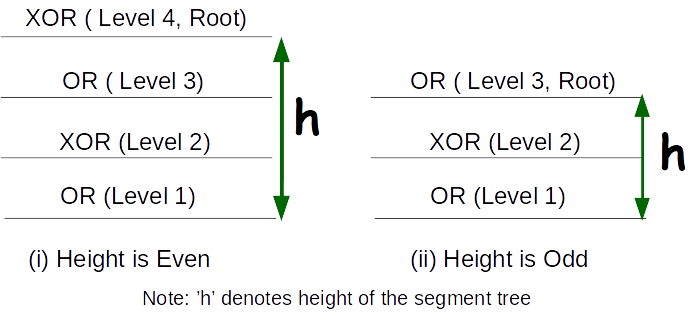# Levelwise Alternating OR and XOR operations in Segment Tree

A Levelwise OR/XOR alternating segment tree is a segment tree, such that at every level the operations OR and XOR alternate. In other words at Level 1 the left and right sub-trees combine together by the OR operation i.e Parent node = Left Child OR Right Child and on Level 2 the left
and right sub-trees combine together by the XOR operation i.e Parent node = Left Child XOR Right Child

Such a type of Segment tree has the following type of structure:The operations (OR) and (XOR) indicate which operation was carried out to merge the child node

Given 2N leaf nodes, the task is to build such a segment tree and print the root node

Examples:

```Input : Leaves = {1, 6, 3, 7, 5, 9, 10, 4}
Output : Value at Root Node = 3
Explanation : The image given above shows the
segment tree corresponding to the given
set leaf nodes.
```

## Recommended: Please try your approach on {IDE} first, before moving on to the solution.

This is a Extension to the Classical Segment Tree where we represent each node as as integer and the parent node is built by first building the left and the right subtrees and then combining the results of the left and the right children into the parent node. This merging of the left and right children into the parent node is done by a consistent operations For example, MIN()/MAX() in Range Minimum Queries, Sum, XOR, OR, AND, etc. By consistent operations, we mean that this operation is performed to merge any node’s left and right child into the parent node by carrying the operation say OP on their results, where OP is a consistent operation.

In this Segment tree, we carry two operations that are -: OR and XOR.

Now we build the Segment tree in a similar fashion as we do in the classical version, but now when we recursively pass the information for the sub-trees we will also pass information regarding the operation to be carried out at that level since these operations alternate levelwise. Its important to note that a parent node when calls its left and right children the same operation information is passed to both the children as they are on the same level.

Lets represent the two operations i.e OR and XOR by 0 and 1 respectively. Then if at Level i we have an OR operation the at Level (i + 1) we will have an XOR operation. There if Level i has 0 as operation then level (i + 1) will have 1 as operation

```Operation at Level (i + 1) = ! (Operation at Level i)
where,
Operation at Level i ∈ {0, 1}
```

The parent child relationship for a segment tree node is shown in the below image:Now, we need to look at the operation to be carried out at root node.If we carefully look at the image then it would be easy to figure out that if the height of the tree is even then the root node is a result of XOR operation of its left and right children else a result of OR operation.

## C++

 `// C++ program to build levelwise OR/XOR alternating ` `// Segment tree ` `#include ` ` `  `using` `namespace` `std; ` ` `  `// A utility function to get the middle index from  ` `// corner indexes. ` `int` `getMid(``int` `s, ``int` `e) { ``return` `s + (e - s) / 2; } ` ` `  `// A recursive function that constructs Segment Tree ` `// for array[ss..se].  ` `// si is index of current node in segment tree st ` `// operation denotes which operation is carried out  ` `// at that level to merge the left and right child.  ` `// It's either 0 or 1. ` `void` `constructSTUtil(``int` `arr[], ``int` `ss, ``int` `se, ``int``* st,  ` `                                  ``int` `si, ``int` `operation) ` `{ ` `    ``// If there is one element in array, store it  ` `    ``// in current node of segment tree and return ` `    ``if` `(ss == se) { ` `        ``st[si] = arr[ss]; ` `        ``return``; ` `    ``} ` ` `  `    ``// If there are more than one elements, then ` `    ``// recur for left and right subtrees and store  ` `    ``// the sum of values in this node ` `    ``int` `mid = getMid(ss, se); ` ` `  `    ``// Build the left and the right subtrees by ` `    ``// using the fact that operation at level  ` `    ``// (i + 1) = ! (operation at level i) ` `    ``constructSTUtil(arr, ss, mid, st, si * 2 + 1, !operation); ` `    ``constructSTUtil(arr, mid + 1, se, st, si * 2 + 2, !operation); ` ` `  `    ``// merge the left and right subtrees by checking  ` `    ``// the operation to  be carried. If operation = 1, ` `    ``// then do OR else XOR ` `    ``if` `(operation == 1) { ` ` `  `        ``// OR operation ` `        ``st[si] = (st[2 * si + 1] | st[2 * si + 2]); ` `    ``} ` `    ``else` `{ ` ` `  `        ``// XOR operation ` `        ``st[si] = (st[2 * si + 1] ^ st[2 * si + 2]); ` `    ``} ` `} ` ` `  `/* Function to construct segment tree from given array.  ` `   ``This function allocates memory for segment tree and  ` `   ``calls constructSTUtil() to fill the allocated memory */` `int``* constructST(``int` `arr[], ``int` `n) ` `{ ` `    ``// Allocate memory for segment tree ` ` `  `    ``// Height of segment tree ` `    ``int` `x = (``int``)(``ceil``(log2(n))); ` ` `  `    ``// Maximum size of segment tree ` `    ``int` `max_size = 2 * (``int``)``pow``(2, x) - 1; ` ` `  `    ``// Allocate memory ` `    ``int``* st = ``new` `int``[max_size]; ` ` `  `    ``// operation = 1(XOR) if Height of tree is ` `    ``// even else it is 0(OR) for the root node ` `    ``int` `operationAtRoot = (x % 2 == 0 ? 0 : 1); ` ` `  `    ``// Fill the allocated memory st ` `    ``constructSTUtil(arr, 0, n - 1, st, 0, operationAtRoot); ` ` `  `    ``// Return the constructed segment tree ` `    ``return` `st; ` `} ` ` `  `// Driver Code ` `int` `main() ` `{ ` ` `  `    ``// leaf nodes ` `    ``int` `leaves[] = { 1, 6, 3, 7, 5, 9, 10, 4 }; ` ` `  `    ``int` `n = ``sizeof``(leaves) / ``sizeof``(leaves); ` ` `  `    ``// Build the segment tree ` `    ``int``* segmentTree = constructST(leaves, n); ` ` `  `    ``// Root node is at index 0 considering ` `    ``// 0-based indexing in segment Tree ` `    ``int` `rootIndex = 0; ` ` `  `    ``// print value at rootIndex ` `    ``cout << ``"Value at Root Node = "` `<< segmentTree[rootIndex]; ` `} `

## Python 3

 `# Python 3 program to build levelwise  ` `# OR/XOR alternating Segment tree ` `import` `math ` ` `  `# A utility function to get the ` `#  middle index from corner indexes. ` `def` `getMid(s, e): ` `    ``return` `s ``+` `(e ``-` `s) ``/``/` `2` ` `  `# A recursive function that constructs ` `# Segment Tree for array[ss..se].  ` `# 'si' is index of current node in segment  ` `# tree 'st', operation denotes which operation  ` `# is carried out at that level to merge the  ` `# left and right child. It's either 0 or 1. ` `def` `constructSTUtil(arr, ss, se, st, si, operation): ` ` `  `    ``# If there is one element in array,  ` `    ``# store it in current node of segment ` `    ``# tree and return ` `    ``if` `(ss ``=``=` `se) : ` `        ``st[si] ``=` `arr[ss] ` `        ``return` `     `  `    ``# If there are more than one elements,  ` `    ``# then recur for left and right subtrees  ` `    ``# and store the sum of values in this node ` `    ``mid ``=` `getMid(ss, se) ` ` `  `    ``# Build the left and the right subtrees by ` `    ``# using the fact that operation at level  ` `    ``# (i + 1) = ! (operation at level i) ` `    ``constructSTUtil(arr, ss, mid, st,  ` `                    ``si ``*` `2` `+` `1``, ``not` `operation) ` `    ``constructSTUtil(arr, mid ``+` `1``, se, st,  ` `                    ``si ``*` `2` `+` `2``, ``not` `operation) ` ` `  `    ``# merge the left and right subtrees  ` `    ``# by checking the operation to be  ` `    ``# carried. If operation = 1, then  ` `    ``# do OR else XOR ` `    ``if` `(operation ``=``=` `1``) : ` ` `  `        ``# OR operation ` `        ``st[si] ``=` `(st[``2` `*` `si ``+` `1``] |  ` `                  ``st[``2` `*` `si ``+` `2``]) ` `     `  `    ``else` `: ` ` `  `        ``# XOR operation ` `        ``st[si] ``=` `(st[``2` `*` `si ``+` `1``] ^  ` `                  ``st[``2` `*` `si ``+` `2``]) ` ` `  `''' Function to construct segment tree  ` `from given array. This function allocates  ` `memory for segment tree and calls  ` `constructSTUtil() to fill the allocated memory '''` `def` `constructST(arr, n): ` ` `  `    ``# Allocate memory for segment tree ` ` `  `    ``# Height of segment tree ` `    ``x ``=` `int``(math.ceil(math.log2(n))) ` ` `  `    ``# Maximum size of segment tree ` `    ``max_size ``=` `2` `*` `int``(``pow``(``2``, x)) ``-` `1` ` `  `    ``# Allocate memory ` `    ``st ``=` `[``0``] ``*` `max_size ` ` `  `    ``# operation = 1(XOR) if Height of tree is ` `    ``# even else it is 0(OR) for the root node ` `    ``operationAtRoot ``=` `(``0` `if` `x ``%` `2` `=``=` `0` `else` `1``) ` ` `  `    ``# Fill the allocated memory st ` `    ``constructSTUtil(arr, ``0``, n ``-` `1``,  ` `                    ``st, ``0``, operationAtRoot) ` ` `  `    ``# Return the constructed segment tree ` `    ``return` `st ` ` `  `# Driver Code ` `if` `__name__ ``=``=` `"__main__"``: ` ` `  `    ``# leaf nodes ` `    ``leaves ``=` `[ ``1``, ``6``, ``3``, ``7``, ``5``, ``9``, ``10``, ``4` `] ` ` `  `    ``n ``=` `len``(leaves) ` ` `  `    ``# Build the segment tree ` `    ``segmentTree ``=` `constructST(leaves, n) ` ` `  `    ``# Root node is at index 0 considering ` `    ``# 0-based indexing in segment Tree ` `    ``rootIndex ``=` `0` ` `  `    ``# print value at rootIndex ` `    ``print``(``"Value at Root Node = "` `,  ` `           ``segmentTree[rootIndex]) ` ` `  `# This code is contributed by ChitraNayal `

Output:

```Value at Root Node = 3
```

Time Complexity for tree construction is O(n). There are total 2n-1 nodes, and value of every node is calculated only once in tree construction.

We can also perform Point Updates in a similar manner. If we get an update to update the leaf at index i of the array leaves then we traverse down the tree to the leaf node and perform the update. While coming back to the root node we build the tree again similar to the build() function by passing the operation to be performed at every level and storing the result of applying that operation on values of its left and right children and storing the result into that node.

Consider the following Segment tree after performing the update,
Leaves = 13
Now the updated segment tree looks like this:Here the nodes in black denote the fact that they were updated

 `// C++ program to build levelwise OR/XOR alternating  ` `// Segment tree (with updates) ` `#include ` ` `  `using` `namespace` `std; ` ` `  `// A utility function to get the middle index from ` `// corner indexes. ` `int` `getMid(``int` `s, ``int` `e) { ``return` `s + (e - s) / 2; } ` ` `  `// A recursive function that constructs Segment Tree  ` `// for array[ss..se]. ` `// si is index of current node in segment tree st ` `// operation denotes which operation is carried out ` `// at that level to merge the left and right child.  ` `// Its either 0 or 1. ` `void` `constructSTUtil(``int` `arr[], ``int` `ss, ``int` `se, ``int``* st, ` `                                  ``int` `si, ``int` `operation) ` `{ ` `    ``// If there is one element in array, store it in  ` `    ``// current node of segment tree and return ` `    ``if` `(ss == se) { ` `        ``st[si] = arr[ss]; ` `        ``return``; ` `    ``} ` ` `  `    ``// If there are more than one elements, then recur ` `    ``// for left and right subtrees and store the sum of  ` `    ``// values in this node ` `    ``int` `mid = getMid(ss, se); ` ` `  `    ``// Build the left and the right subtrees by using ` `    ``// the fact that operation at level (i + 1) = !  ` `    ``// (operation at level i) ` `    ``constructSTUtil(arr, ss, mid, st, si * 2 + 1, !operation); ` `    ``constructSTUtil(arr, mid + 1, se, st, si * 2 + 2, !operation); ` ` `  `    ``// merge the left and right subtrees by checking ` `    ``// the operation to be carried. If operation = 1,  ` `    ``// then do OR else XOR ` `    ``if` `(operation == 1) { ` ` `  `        ``// OR operation ` `        ``st[si] = (st[2 * si + 1] | st[2 * si + 2]); ` `    ``} ` `    ``else` `{ ` `        ``// XOR operation ` `        ``st[si] = (st[2 * si + 1] ^ st[2 * si + 2]); ` `    ``} ` `} ` ` `  `/* A recursive function to update the nodes which have the given  ` `   ``index in their range. The following are parameters ` `    ``st, si, ss and se are same as getSumUtil() ` `    ``i    --> index of the element to be updated. This index is  ` `             ``in input array. ` `   ``val --> Value to be assigned to arr[i] */` `void` `updateValueUtil(``int``* st, ``int` `ss, ``int` `se, ``int` `i, ` `                     ``int` `val, ``int` `si, ``int` `operation) ` `{ ` `    ``// Base Case: If the input index lies outside  ` `    ``//  this segment ` `    ``if` `(i < ss || i > se) ` `        ``return``; ` ` `  `    ``// If the input index is in range of this node, ` `    ``// then update the value of the node and its  ` `    ``// children ` ` `  `    ``// leaf node ` `    ``if` `(ss == se && ss == i) { ` `        ``st[si] = val; ` `        ``return``; ` `    ``} ` ` `  `    ``int` `mid = getMid(ss, se); ` ` `  `    ``// Update the left and the right subtrees by ` `    ``// using the fact that operation at level  ` `    ``// (i + 1) = ! (operation at level i) ` `    ``updateValueUtil(st, ss, mid, i, val, 2 * si + 1, !operation); ` `    ``updateValueUtil(st, mid + 1, se, i, val, 2 * si + 2, !operation); ` ` `  `    ``// merge the left and right subtrees by checking ` `    ``// the operation to be carried. If operation = 1,  ` `    ``// then do OR else XOR ` `    ``if` `(operation == 1) { ` `        `  `        ``// OR operation ` `        ``st[si] = (st[2 * si + 1] | st[2 * si + 2]); ` `    ``} ` `    ``else` `{ ` ` `  `        ``// XOR operation ` `        ``st[si] = (st[2 * si + 1] ^ st[2 * si + 2]); ` `    ``} ` `} ` ` `  `// The function to update a value in input array and  ` `// segment tree. It uses updateValueUtil() to update the  ` `// value in segment tree ` `void` `updateValue(``int` `arr[], ``int``* st, ``int` `n, ``int` `i, ``int` `new_val) ` `{ ` `    ``// Check for erroneous input index ` `    ``if` `(i < 0 || i > n - 1) { ` `        ``printf``(``"Invalid Input"``); ` `        ``return``; ` `    ``} ` ` `  `    ``// Height of segment tree ` `    ``int` `x = (``int``)(``ceil``(log2(n))); ` ` `  `    ``// operation = 1(XOR) if Height of tree is ` `    ``// even else it is 0(OR) for the root node ` `    ``int` `operationAtRoot = (x % 2 == 0 ? 0 : 1); ` ` `  `    ``arr[i] = new_val; ` ` `  `    ``// Update the values of nodes in segment tree ` `    ``updateValueUtil(st, 0, n - 1, i, new_val, 0, operationAtRoot); ` `} ` ` `  `/* Function to construct segment tree from given array. ` `   ``This function allocates memory for segment tree and  ` `   ``calls constructSTUtil() to fill the allocated memory */` `int``* constructST(``int` `arr[], ``int` `n) ` `{ ` `    ``// Allocate memory for segment tree ` ` `  `    ``// Height of segment tree ` `    ``int` `x = (``int``)(``ceil``(log2(n))); ` ` `  `    ``// Maximum size of segment tree ` `    ``int` `max_size = 2 * (``int``)``pow``(2, x) - 1; ` ` `  `    ``// Allocate memory ` `    ``int``* st = ``new` `int``[max_size]; ` ` `  `    ``// operation = 1(XOR) if Height of tree is  ` `    ``// even else it is 0(OR) for the root node ` `    ``int` `operationAtRoot = (x % 2 == 0 ? 0 : 1); ` ` `  `    ``// Fill the allocated memory st ` `    ``constructSTUtil(arr, 0, n - 1, st, 0, operationAtRoot); ` ` `  `    ``// Return the constructed segment tree ` `    ``return` `st; ` `} ` ` `  `// Driver Code ` `int` `main() ` `{ ` `    ``int` `leaves[] = { 1, 6, 3, 7, 5, 9, 10, 4 }; ` `    ``int` `n = ``sizeof``(leaves) / ``sizeof``(leaves); ` ` `  `    ``// Build the segment tree ` `    ``int``* segmentTree = constructST(leaves, n); ` ` `  `    ``// Root node is at index 0 considering ` `    ``// 0-based indexing in segment Tree ` `    ``int` `rootIndex = 0; ` ` `  `    ``// print old value at rootIndex ` `    ``cout << ``"Old Value at Root Node = "`  `         ``<< segmentTree[rootIndex] << endl; ` ` `  `    ``// perform update leaves = 13 ` `    ``updateValue(leaves, segmentTree, n, 0, 13); ` ` `  `    ``cout << ``"New Value at Root Node = "`  `         ``<< segmentTree[rootIndex]; ` `    ``return` `0; ` `} `

Output:

```Old Value at Root Node = 3
New Value at Root Node = 11
```

The time complexity of update is also O(Logn). To update a leaf value, we process one node at every level and number of levels is O(Logn).

GeeksforGeeks has prepared a complete interview preparation course with premium videos, theory, practice problems, TA support and many more features. Please refer Placement 100 for details

My Personal Notes arrow_drop_upCheck out this Author's contributed articles.

If you like GeeksforGeeks and would like to contribute, you can also write an article using contribute.geeksforgeeks.org or mail your article to contribute@geeksforgeeks.org. See your article appearing on the GeeksforGeeks main page and help other Geeks.

Please Improve this article if you find anything incorrect by clicking on the "Improve Article" button below.